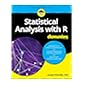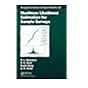Normal view MARC view ISBD view

Basics of matrix algebra for statistics with R

Material type:BookSeries: Chapman & Hall/CRC The R series. Publisher: Boca Raton CRC Press 2016Description: xviii, 226 p.ISBN: 9781498712361 .DDC classification: 519.5028553 Summary: Basics of Matrix Algebra for Statistics with R provides a guide to elementary matrix algebra sufficient for undertaking specialized courses, such as multivariate data analysis and linear models. It also covers advanced topics, such as generalized inverses of singular and rectangular matrices and manipulation of partitioned matrices, for those who want to delve deeper into the subject. The book introduces the definition of a matrix and the basic rules of addition, subtraction, multiplication, and inversion. Later topics include determinants, calculation of eigenvectors and eigenvalues, and differentiation of linear and quadratic forms with respect to vectors. The text explores how these concepts arise in statistical techniques, including principal component analysis, canonical correlation analysis, and linear modeling. In addition to the algebraic manipulation of matrices, the book presents numerical examples that illustrate how to perform calculations by hand and using R. Many theoretical and numerical exercises of varying levels of difficulty aid readers in assessing their knowledge of the material. Outline solutions at the back of the book enable readers to verify the techniques required and obtain numerical answers. Avoiding vector spaces and other advanced mathematics, this book shows how to manipulate matrices and perform numerical calculations in R. It prepares readers for higher-level and specialized studies in statistics. (https://www.crcpress.com/Basics-of-Matrix-Algebra-for-Statistics-with-R/Fieller/9781498712361)
Tags from this library: No tags from this library for this title.
average rating: 0.0 (0 votes)
Item type Current location Collection Call number Status Date due Barcode
Books Vikram Sarabhai Library
General Stacks
Non-fiction 519.5028553 F4B2 (Browse shelf) Available 191146
Browsing Vikram Sarabhai Library Shelves , Shelving location: General Stacks , Collection code: Non-fiction Close shelf browser519.502855133 S2S8 Statistical analysis with R for dummies 519.502855133 V2P9 Python data science handbook: essential tools for working with data 519.502855133 V3U8-2014 Using R for introductory statistics 519.5028553 F4B2 Basics of matrix algebra for statistics with R 519.50285536 A6M6-2015 Modern business statistics with Microsoft Office Excel 519.52 C4I6 An introduction to model-based survey sampling with applications 519.52 C4M2 Maximum likelihood estimation for sample surveys

Introduction
• Objectives
• Guide to Notation
• An Outline Guide to R
• Inputting Data to R
• Summary of Matrix Operators in R
• Examples of R Commands

Vectors and Matrices
• Vectors
• Matrices
• Matrix Arithmetic
• Transpose and Trace of Sums and Products
• Special Matrices
• Partitioned Matrices
• Algebraic Manipulation of matrices
• Useful Tricks
• Linear and Quadratic Forms
• Creating Matrices in R
• Matrix Arithmetic in R
• Initial Statistical Applications

Rank of Matrices
• Introduction and Definitions
• Rank Factorization
• Rank Inequalities
• Rank in Statistics

Determinants
• Introduction and Definitions
• Implementation in R
• Properties of Determinants
• Orthogonal Matrices
• Determinants of Partitioned Matrices
• A Key Property of Determinants

Inverses
• Introduction and Definitions
• Properties
• Implementation in R
• Inverses of Patterned Matrices
• Inverses of Partitioned Matrices
• General Formulae
• Initial Applications Continued

Eigenanalysis of Real Symmetric Matrices
• Introduction and Definitions
• Eigenvectors
• Implementation in R
• Properties of Eigenanalyses
• A Key Statistical Application: PCA
• Matrix Exponential
• Decompositions
• Eigenanalysis of Matrices with Special Structures
• Summary of Key Results

Vector and Matrix Calculus
• Introduction
• Differentiation of a Scalar with Respect to a Vector
• Differentiation of a Scalar with Respect to a Matrix
• Differentiation of a Vector with Respect to a Vector
• Differentiation of a Matrix with Respect to a Scalar
• Use of Eigenanalysis in Constrained Optimization

Further Topics
• Introduction
• Further Matrix Decompositions
• Generalized Inverses
• Kronecker Products and the Vec Operator

Key Applications to Statistics
• Introduction
• The Multivariate Normal Distribution
• Principal Component Analysis
• Linear Discriminant Analysis
• Canonical Correlation Analysis
• Classical Scaling
• Linear Models

Outline Solutions to Exercises

Basics of Matrix Algebra for Statistics with R provides a guide to elementary matrix algebra sufficient for undertaking specialized courses, such as multivariate data analysis and linear models. It also covers advanced topics, such as generalized inverses of singular and rectangular matrices and manipulation of partitioned matrices, for those who want to delve deeper into the subject.

The book introduces the definition of a matrix and the basic rules of addition, subtraction, multiplication, and inversion. Later topics include determinants, calculation of eigenvectors and eigenvalues, and differentiation of linear and quadratic forms with respect to vectors. The text explores how these concepts arise in statistical techniques, including principal component analysis, canonical correlation analysis, and linear modeling.

In addition to the algebraic manipulation of matrices, the book presents numerical examples that illustrate how to perform calculations by hand and using R. Many theoretical and numerical exercises of varying levels of difficulty aid readers in assessing their knowledge of the material. Outline solutions at the back of the book enable readers to verify the techniques required and obtain numerical answers.

Avoiding vector spaces and other advanced mathematics, this book shows how to manipulate matrices and perform numerical calculations in R. It prepares readers for higher-level and specialized studies in statistics.

(https://www.crcpress.com/Basics-of-Matrix-Algebra-for-Statistics-with-R/Fieller/9781498712361)

There are no comments for this item.